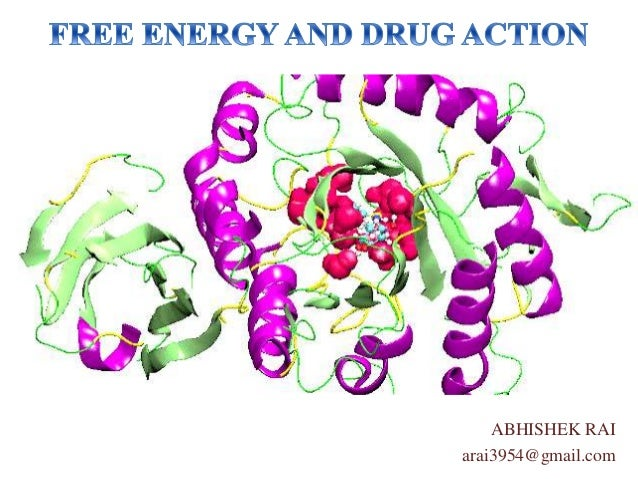Successfully reported this slideshow.Upcoming SlideShare
×

# Free energy and drug interaction

789 views

Published on

definitions of bioenergetics, system, surroundings, universe, isolated closed and open systems, laws of thermodynamics, enthalpy, entropy, free energy, gibb's free energy, exergonic and endergonic reactions, thermodyanamics and cell functions, drug- receptor interactions.

Published in: Health & Medicine
• Full Name
Comment goes here.

Are you sure you want to Yes No• Be the first to like this

### Free energy and drug interaction

1. 1. ABHISHEK RAI arai3954@gmail.com
2. 2. • The quantitative study of energy relationships and energy conversions in living system. • All biological transformations obey laws of thermodynamics. BIOENERGETICS: • part of universe in which observations are being made. It includes all the reactants and products present, the solvent that contains them and the immediate atmosphere. SYSTEM: • universe apart from system.SURROUNDINGS: • System and surrounding together.UNIVERSE:
3. 3. • System that neither exchanges matter nor its energy from surroundings. ISOLATED SYSTEM • System that exchanges energy but does not matter with surroundings. CLOSED SYSTEM • System that exchanges both energy and matter with surroundings.OPEN SYSTEM
4. 4. • If two systems are separately in thermal equilibrium with third, they will also be in thermal equilibrium. ZEROTH LAW OF THERMODYNAMIC S • In any physical or chemical change, the total amount of energy in the universe remains constant, although the form of the energy may change. FIRST LAW OF THERMODYNAMIC S • The total entropy of the universe is continually increasing. SECOND LAW OF THERMODYNAMIC S
5. 5. • Heat content of the system. Denoted by ‘H’. • Reflects the no. and kinds of chemical bonds in the reactants and products. ENTHALPY • Quantitative expression for randomness or disorder in the system. • Denoted by ‘S’. ENTROPY • Free energy content of the system. Denoted by ‘G’. • Expresses the amount of energy capable of doing workduring a reaction at constant temperature and pressure FREE ENERGY
6. 6. Free energy, G = H – TS. The free energy change, ΔG = ΔH – TΔS. where, ΔH = the enthalpy change, reflecting the kinds and numbers of chemical bonds and non-covalent interactions broken and formed. ΔS = the entropy change, describing the change in the system’s randomness. For a process to occur spontaneously, ΔG must be negative.
7. 7. • The process having negative value of ΔG. • Spontaneous in nature. • ΔG = ΔH – TΔS < 0 EXERGONIC REACTIONS • The process having positive value of ΔG. • Are not spontaneous. • ΔG = ΔH – TΔS > 0 ENDERGONIC REACTIONS
8. 8. •Cellular functions depend largely on macromolecules such as proteins and nucleic acids. •ΔG for there formation have positive values: the molecules are less stable and more highly ordered then the mixture of their monomeric constituents. •These thermodynamically unfavorable, endergonic reactions are carried out by coupling them with exergonic reactions (usually hydrolysis of ATP): the sum of free energy change is negative , eg, Amino acids --------------> polymer ΔG1 is positive. --Ᵽ--Ᵽ ----------------> --Ᵽ + Ᵽ ΔG2 is negative. •For such coupled reactions sum of ΔG1 and ΔG2 is negative, ie the overall process is exergonic.
9. 9. Drug-receptor interaction can be written as : L:Sl + R:Sr + Sbulk <=> L*:R*:Slr + S*bulk where, L:Sl = free ligand in solution surrounded by perturbed solvation shell R:Sr = free receptor together with a perturbed solvation shell, Sbulk = bulk solvent, L*:R*:Slr = complex ofligand and receptor and a perturbed solution shell, and, S*bulk = bulk solvent.
10. 10. The position of equilibrium and affinity of ligand are defined by ‘Free Energy Difference’(ΔG). ΔG = RT ln Kd this can be used to determine binding affinity by ΔG. ΔG itself can be calculated from, ΔG = ΔH – TΔS
11. 11. The overall free energy can be calculated by formula such as, ΔG = ΔGt+r + ΔGr + ΔGx + Δgconf where, ΔGt+r = cost of binding the ligand to the receptor, ΔGr = cost of restricting internal rotations, ΔGx = sum of contributions of individual functional groups X, including weak intermolecular bonds such as hydrogen bonds and free energy change associated with solvent reorganization(the hydrophobic effect), and Δgconf = energy penalty for binding high energy conformer.
12. 12. •Nelson D L, Cox M M, ‘Lehninger’s Principles of Biochemistry’ edition 6th 2007. •Murray R K, Granner D K, Rodwell V W, ‘Harper’s Illustrated Biochemistry’ edition 27th Mc Graw Hill, 2006. •Larsen P K, Liljefors T, & Madsen U ‘Textbook of Drug Design and Discovery’ edition 3rd, Tylor and Fransics, 2002.